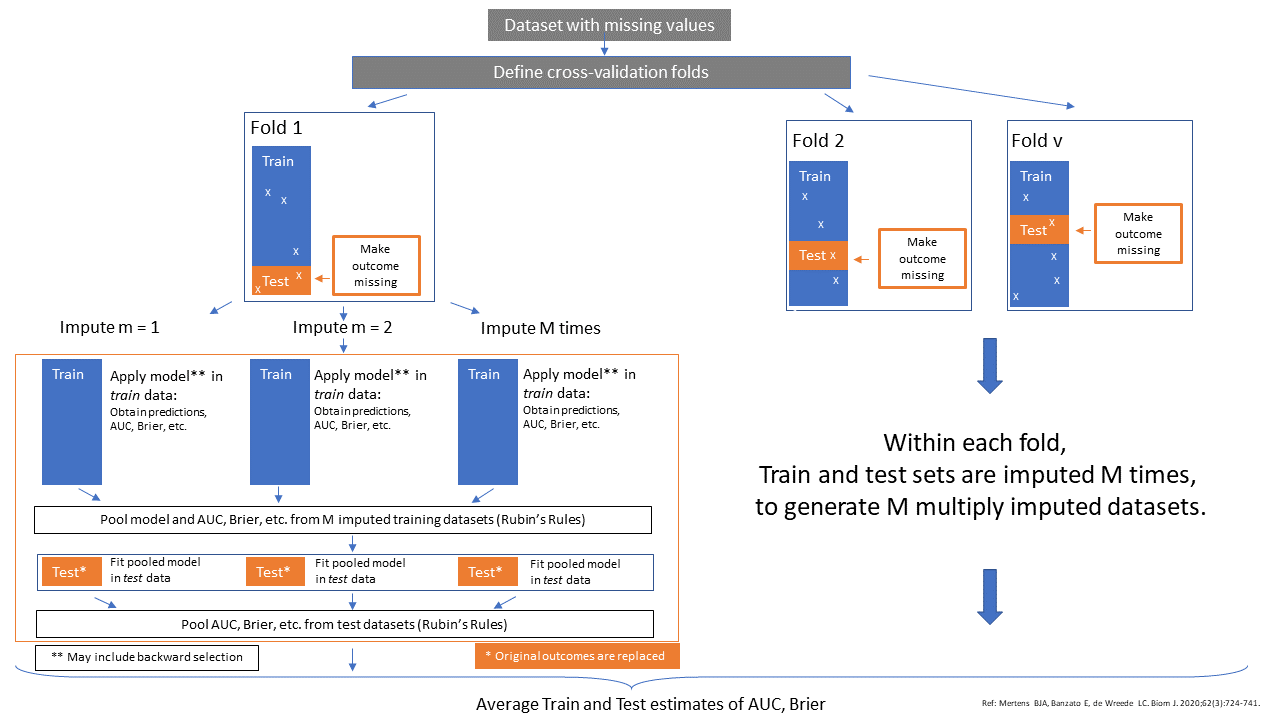Introduction

This page contains information of the cv_MI_RR method that combines Multiple Imputation with Cross-validation for the validation of logistic prediction models. This cross-validation method is based on the paper ofs Mertens BJ and Miles A. The cv_MI_RR method is implemented in the function psfmi_validate. An explanation and examples of how to use the methods can be found below.

Method cv_MI_RR

The method cv_MI_RR uses multiple imputation within the cross-validation definition. The pooled model is analyzed in the training data and subsequently tested in the test data. The method can be performed in combination with backward selection of the pooled model in the training set and subsequently testing the performance of the pooled model in the test set. The method can only be performed when the outcome data is complete.

How these steps work is visualized in the Figure below.Schematic overview of the cv_MI_RR method

Examples

Method cv_MI_RR

To run the cv_MI_RR method use:

library(psfmi)
pool_lr <- psfmi_lr(data=lbpmilr, formula = Chronic ~ Pain + JobDemands + rcs(Tampascale, 3) +
factor(Satisfaction) + Smoking, p.crit = 1, direction="BW",
nimp=5, impvar="Impnr", method="D1")

set.seed(200)
res_cv <- psfmi_validate(pool_lr, val_method = "cv_MI_RR", data_orig = lbp_orig, folds = 3,
p.crit=1, BW=FALSE,  nimp_mice = 5, miceImp = miceImp, printFlag = FALSE)
##
## fold 1
##
## fold 2
##
## fold 3
res_cv
## $stats ## Train Test ## AUC 0.8977368 0.8614725 ## Brier scaled 0.4750403 0.2930774 ## Rsq 0.5777454 0.4391906 ## ##$slope
## Intercept     Slope
## 0.1256736 0.9104715

Back to Examples

Method cv_MI_RR including BW selection

To run the cv_MI_RR method including backward selection:

library(psfmi)
pool_lr <- psfmi_lr(data=lbpmilr, formula = Chronic ~ Pain + JobDemands + rcs(Tampascale, 3) +
factor(Satisfaction) + Smoking, p.crit = 1, direction="BW",
nimp=5, impvar="Impnr", method="D1")

set.seed(200)
res_cv <- psfmi_validate(pool_lr, val_method = "cv_MI_RR", data_orig = lbp_orig, folds = 3,
p.crit=0.05, BW=TRUE, nimp_mice = 5, miceImp = miceImp, printFlag = FALSE)
##
## fold 1
## Removed at Step 1 is - JobDemands
## Removed at Step 2 is - Smoking
## Removed at Step 3 is - rcs(Tampascale,3)
##
## Selection correctly terminated,
## No more variables removed from the model
##
## fold 2
## Removed at Step 1 is - JobDemands
## Removed at Step 2 is - Smoking
## Removed at Step 3 is - rcs(Tampascale,3)
##
## Selection correctly terminated,
## No more variables removed from the model
##
## fold 3
## Removed at Step 1 is - JobDemands
## Removed at Step 2 is - Smoking
## Removed at Step 3 is - rcs(Tampascale,3)
## Removed at Step 4 is - factor(Satisfaction)
##
## Selection correctly terminated,
## No more variables removed from the model
res_cv
## $stats ## Train Test ## AUC 0.8796046 0.8261287 ## Brier scaled 0.4580478 0.2760269 ## Rsq 0.5328784 0.3955043 ## ##$slope
##  Intercept      Slope
## -0.0182750  0.8884874

Back to Examples